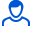Content: 00062.png (77.92 KB)

Positive responses: 0
Negative responses: 0

Sold: 16
Refunds: 0Loyalty discount! If the total amount of your purchases from the seller more than:

 \$1 the discount is 10% \$5 the discount is 30%

Determine the quality factor L-R-C of a serial electric circuit containing a periodic emf, if the coil inductance is L = 2.5 H, the active resistance of the circuit is R = 12.5 Ohms, and the maximum voltage value across the resistor Um = 50 V is reached at a frequency of 9.28 Hz. Consider attenuation small. Determine the resonant amplitude of the current oscillations. Write the differential equation of charge oscillations on the plates of the capacitor and its solution in the steady state.
Task 00062. Detailed solution with a brief record of the conditions, formulas and laws used in the decision, the conclusion of the calculation formula and the answer.
If you have any questions about the decision, write. I will try to help. File in image format.
No feedback yet